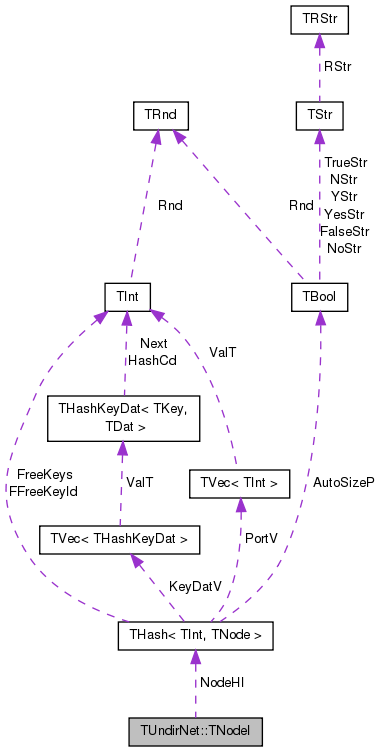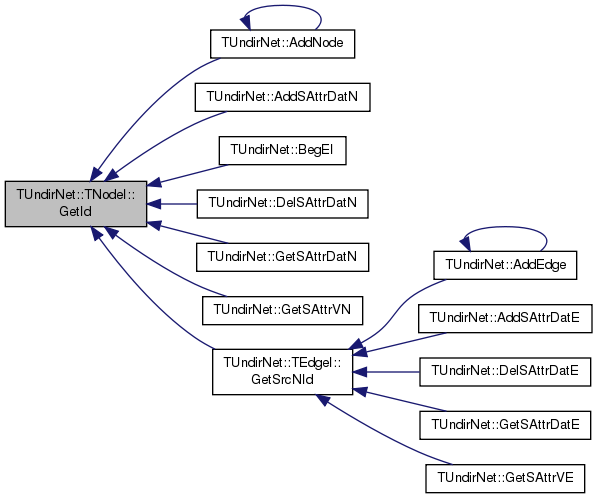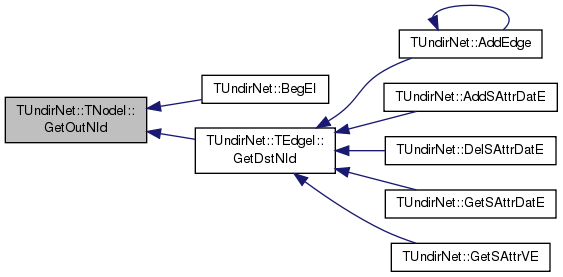SNAP Library 6.0, Developer Reference  2020-12-09 16:24:20 SNAP, a general purpose, high performance system for analysis and manipulation of large networks
TUndirNet::TNodeI Class Reference

Node iterator. Only forward iteration (operator++) is supported. More...

`#include <network.h>`

Collaboration diagram for TUndirNet::TNodeI:[legend]

## Public Member Functions

TNodeI ()

TNodeI (const THashIter &NodeHIter)

TNodeI (const TNodeI &NodeI)

TNodeIoperator= (const TNodeI &NodeI)

TNodeIoperator++ (int)
Increment iterator. More...

TNodeIoperator-- (int)
Decrement iterator. More...

bool operator< (const TNodeI &NodeI) const

bool operator== (const TNodeI &NodeI) const

int GetId () const
Returns ID of the current node. More...

int GetDeg () const
Returns degree of the current node. More...

int GetInDeg () const
Returns in-degree of the current node (returns same as value GetDeg() since the network is undirected). More...

int GetOutDeg () const
Returns out-degree of the current node (returns same as value GetDeg() since the network is undirected). More...

void SortNIdV ()
Sorts the adjacency lists of the current node. More...

int GetInNId (const int &NodeN) const
Returns ID of NodeN-th in-node (the node pointing to the current node). More...

int GetOutNId (const int &NodeN) const
Returns ID of NodeN-th out-node (the node the current node points to). More...

int GetNbrNId (const int &NodeN) const
Returns ID of NodeN-th neighboring node. More...

bool IsInNId (const int &NId) const
Tests whether node with ID NId points to the current node. More...

bool IsOutNId (const int &NId) const
Tests whether the current node points to node with ID NId. More...

bool IsNbrNId (const int &NId) const
Tests whether node with ID NId is a neighbor of the current node. More...

## Private Types

typedef THash< TInt, TNode >::TIter THashIter

THashIter NodeHI

class TUndirNet

## Detailed Description

Node iterator. Only forward iteration (operator++) is supported.

Definition at line 3433 of file network.h.

## Member Typedef Documentation

 typedef THash::TIter TUndirNet::TNodeI::THashIter
private

Definition at line 3435 of file network.h.

## Constructor & Destructor Documentation

 TUndirNet::TNodeI::TNodeI ( )
inline

Definition at line 3438 of file network.h.

3438 : NodeHI() { }
THashIter NodeHI
Definition: network.h:3436
 TUndirNet::TNodeI::TNodeI ( const THashIter & NodeHIter )
inline

Definition at line 3439 of file network.h.

3439 : NodeHI(NodeHIter) { }
THashIter NodeHI
Definition: network.h:3436
 TUndirNet::TNodeI::TNodeI ( const TNodeI & NodeI )
inline

Definition at line 3440 of file network.h.

3440 : NodeHI(NodeI.NodeHI) { }
THashIter NodeHI
Definition: network.h:3436

## Member Function Documentation

 int TUndirNet::TNodeI::GetDeg ( ) const
inline

Returns degree of the current node.

Definition at line 3455 of file network.h.

3455 { return NodeHI.GetDat().GetDeg(); }
THashIter NodeHI
Definition: network.h:3436
 int TUndirNet::TNodeI::GetId ( ) const
inline

Returns ID of the current node.

Definition at line 3453 of file network.h.

3453 { return NodeHI.GetDat().GetId(); }
THashIter NodeHI
Definition: network.h:3436

Here is the caller graph for this function:int TUndirNet::TNodeI::GetInDeg ( ) const
inline

Returns in-degree of the current node (returns same as value GetDeg() since the network is undirected).

Definition at line 3457 of file network.h.

3457 { return NodeHI.GetDat().GetInDeg(); }
THashIter NodeHI
Definition: network.h:3436
 int TUndirNet::TNodeI::GetInNId ( const int & NodeN ) const
inline

Returns ID of NodeN-th in-node (the node pointing to the current node).

Range of NodeN: 0 <= NodeN < GetInDeg(). Since the graph is undirected GetInNId(), GetOutNId() and GetNbrNId() all give the same output.

Definition at line 3466 of file network.h.

3466 { return NodeHI.GetDat().GetInNId(NodeN); }
THashIter NodeHI
Definition: network.h:3436
 int TUndirNet::TNodeI::GetNbrNId ( const int & NodeN ) const
inline

Returns ID of NodeN-th neighboring node.

Range of NodeN: 0 <= NodeN < GetNbrDeg(). Since the graph is undirected GetInNId(), GetOutNId() and GetNbrNId() all give the same output.

Definition at line 3476 of file network.h.

3476 { return NodeHI.GetDat().GetNbrNId(NodeN); }
THashIter NodeHI
Definition: network.h:3436
 int TUndirNet::TNodeI::GetOutDeg ( ) const
inline

Returns out-degree of the current node (returns same as value GetDeg() since the network is undirected).

Definition at line 3459 of file network.h.

Referenced by TUndirNet::BegEI(), and TUndirNet::TEdgeI::operator++().

3459 { return NodeHI.GetDat().GetOutDeg(); }
THashIter NodeHI
Definition: network.h:3436

Here is the caller graph for this function:int TUndirNet::TNodeI::GetOutNId ( const int & NodeN ) const
inline

Returns ID of NodeN-th out-node (the node the current node points to).

Range of NodeN: 0 <= NodeN < GetOutDeg(). Since the graph is undirected GetInNId(), GetOutNId() and GetNbrNId() all give the same output.

Definition at line 3471 of file network.h.

Referenced by TUndirNet::BegEI(), and TUndirNet::TEdgeI::GetDstNId().

3471 { return NodeHI.GetDat().GetOutNId(NodeN); }
THashIter NodeHI
Definition: network.h:3436

Here is the caller graph for this function:bool TUndirNet::TNodeI::IsInNId ( const int & NId ) const
inline

Tests whether node with ID NId points to the current node.

Definition at line 3478 of file network.h.

3478 { return NodeHI.GetDat().IsInNId(NId); }
THashIter NodeHI
Definition: network.h:3436
 bool TUndirNet::TNodeI::IsNbrNId ( const int & NId ) const
inline

Tests whether node with ID NId is a neighbor of the current node.

Definition at line 3482 of file network.h.

3482 { return NodeHI.GetDat().IsNbrNId(NId); }
THashIter NodeHI
Definition: network.h:3436
 bool TUndirNet::TNodeI::IsOutNId ( const int & NId ) const
inline

Tests whether the current node points to node with ID NId.

Definition at line 3480 of file network.h.

3480 { return NodeHI.GetDat().IsOutNId(NId); }
THashIter NodeHI
Definition: network.h:3436
 TNodeI& TUndirNet::TNodeI::operator++ ( int )
inline

Increment iterator.

Definition at line 3444 of file network.h.

3444 { NodeHI++; return *this; }
THashIter NodeHI
Definition: network.h:3436
 TNodeI& TUndirNet::TNodeI::operator-- ( int )
inline

Decrement iterator.

Definition at line 3446 of file network.h.

3446 { NodeHI--; return *this; }
THashIter NodeHI
Definition: network.h:3436
 bool TUndirNet::TNodeI::operator< ( const TNodeI & NodeI ) const
inline

Definition at line 3449 of file network.h.

References NodeHI.

3449 { return NodeHI < NodeI.NodeHI; }
THashIter NodeHI
Definition: network.h:3436
 TNodeI& TUndirNet::TNodeI::operator= ( const TNodeI & NodeI )
inline

Definition at line 3441 of file network.h.

References NodeHI.

3441 { NodeHI = NodeI.NodeHI; return *this; }
THashIter NodeHI
Definition: network.h:3436
 bool TUndirNet::TNodeI::operator== ( const TNodeI & NodeI ) const
inline

Definition at line 3450 of file network.h.

References NodeHI.

3450 { return NodeHI == NodeI.NodeHI; }
THashIter NodeHI
Definition: network.h:3436
 void TUndirNet::TNodeI::SortNIdV ( )
inline

Sorts the adjacency lists of the current node.

Definition at line 3461 of file network.h.

3461 { NodeHI.GetDat().SortNIdV(); }
THashIter NodeHI
Definition: network.h:3436

## Friends And Related Function Documentation

 friend class TUndirNet
friend

Definition at line 3483 of file network.h.

## Member Data Documentation

 THashIter TUndirNet::TNodeI::NodeHI
private

Definition at line 3436 of file network.h.

Referenced by TUndirNet::GetEI(), operator<(), operator=(), and operator==().

The documentation for this class was generated from the following file: